# Inductors and Calculus

## Chapter 15 - Inductors

Inductors do not have a stable “resistance” as conductors do. However, there is a definite mathematical relationship between voltage and current for an inductor, as follows: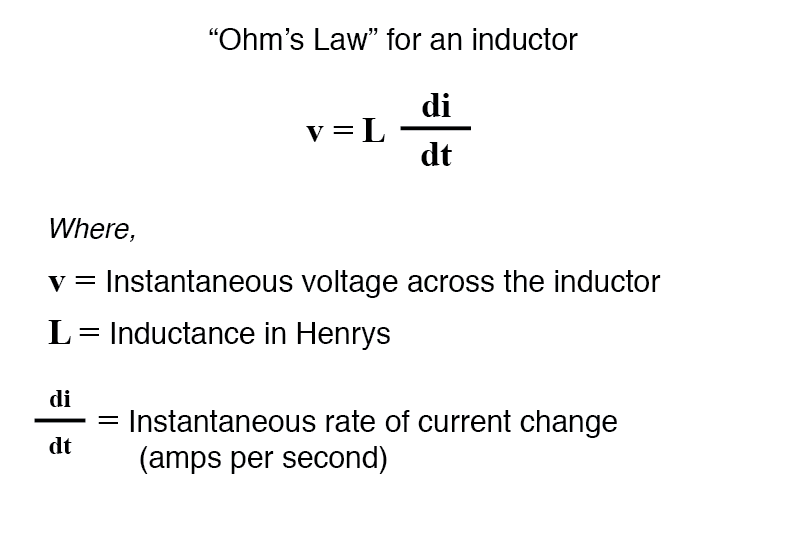You should recognize the form of this equation from the capacitor chapter. It relates one variable (in this case, inductor voltage drop) to a rate of change of another variable (in this case, inductor current). Both voltage (v) and rate of current change (di/dt) are instantaneous: that is, in relation to a specific point in time, thus the lower-case letters “v” and “i”. As with the capacitor formula, it is convention to express instantaneous voltage as v rather than e, but using the latter designation would not be wrong. Current rate-of-change (di/dt) is expressed in units of amps per second, a positive number representing an increase and a negative number representing a decrease.

Like a capacitor, an inductor’s behavior is rooted in the variable of time. Aside from any resistance intrinsic to an inductor’s wire coil (which we will assume is zero for the sake of this section), the voltage dropped across the terminals of an inductor is purely related to how quickly its current changes over time.

Suppose we were to connect a perfect inductor (one having zero ohms of wire resistance) to a circuit where we could vary the amount of current through it with a potentiometer connected as a variable resistor: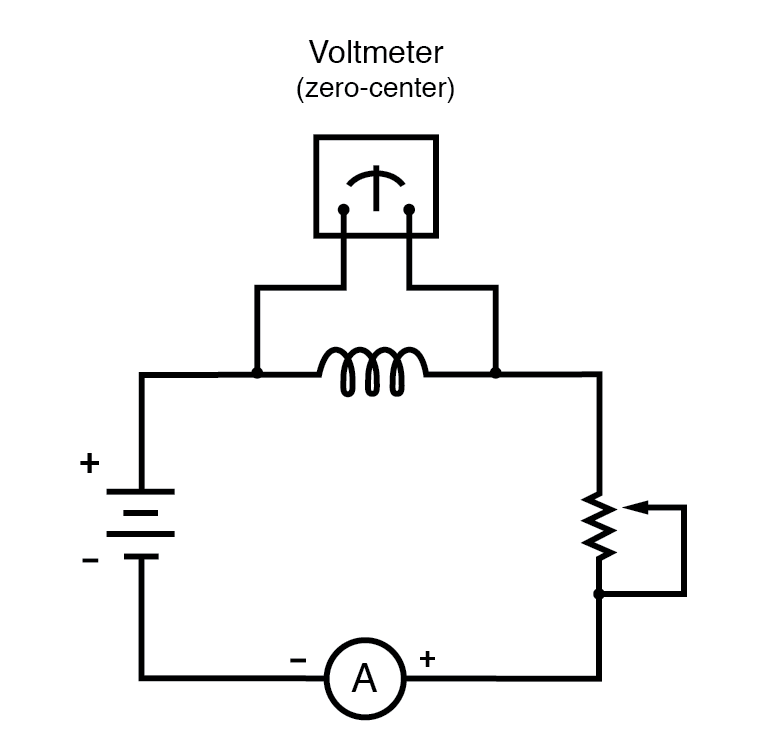If the potentiometer mechanism remains in a single position (wiper is stationary), the series-connected ammeter will register a constant (unchanging) current, and the voltmeter connected across the inductor will register 0 volts. In this scenario, the instantaneous rate of current change (di/dt) is equal to zero, because the current is stable. The equation tells us that with 0 amps per second change for a di/dt, there must be zero instantaneous voltage (v) across the inductor. From a physical perspective, with no current change, there will be a steady magnetic field generated by the inductor. With no change in magnetic flux (dΦ/dt = 0 Webers per second), there will be no voltage dropped across the length of the coil due to induction.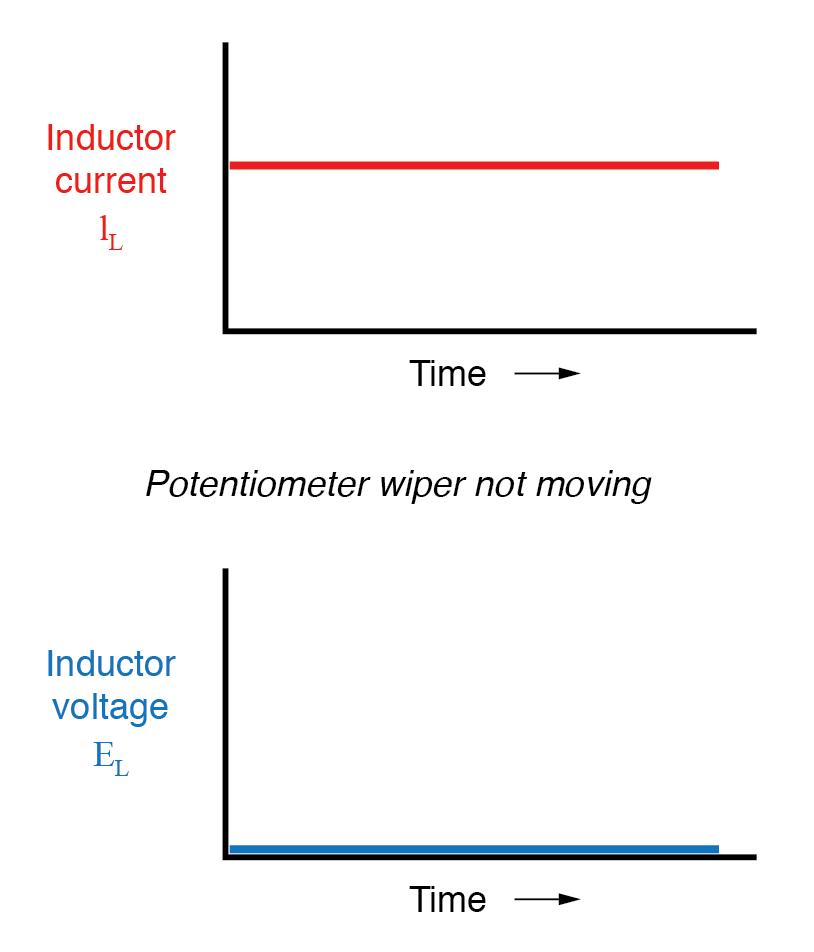If we move the potentiometer wiper slowly in the “up” direction, its resistance from end to end will slowly decrease. This has the effect of increasing current in the circuit, so the ammeter indication should be increasing at a slow rate: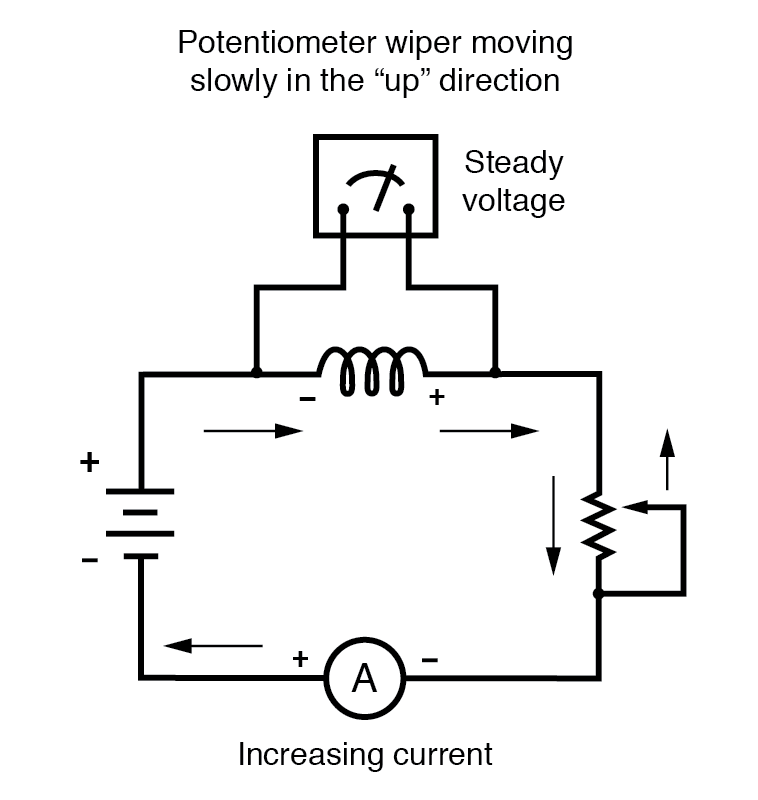Assuming that the potentiometer wiper is being moved such that the rate of current increase through the inductor is steady, the di/dt term of the formula will be a fixed value. This fixed value, multiplied by the inductor’s inductance in Henrys (also fixed), results in a fixed voltage of some magnitude. From a physical perspective, the gradual increase in current results in a magnetic field that is likewise increasing. This gradual increase in magnetic flux causes a voltage to be induced in the coil as expressed by Michael Faraday’s induction equation e = N(dΦ/dt). This self-induced voltage across the coil, as a result of a gradual change in current magnitude through the coil, happens to be of a polarity that attempts to oppose the change in current. In other words, the induced voltage polarity resulting from an increase in current will be oriented in such a way as to push against the direction of current, to try to keep the current at its former magnitude. This phenomenon exhibits a more general principle of physics known as Lenz’s Law, which states that an induced effect will always be opposed to the cause producing it.

### Inductor Current, Voltage vs Time

In this scenario, the inductor will be acting as a load, with the negative side of the induced voltage on the end where electrons are entering, and the positive side of the induced voltage on the end where electrons are exiting.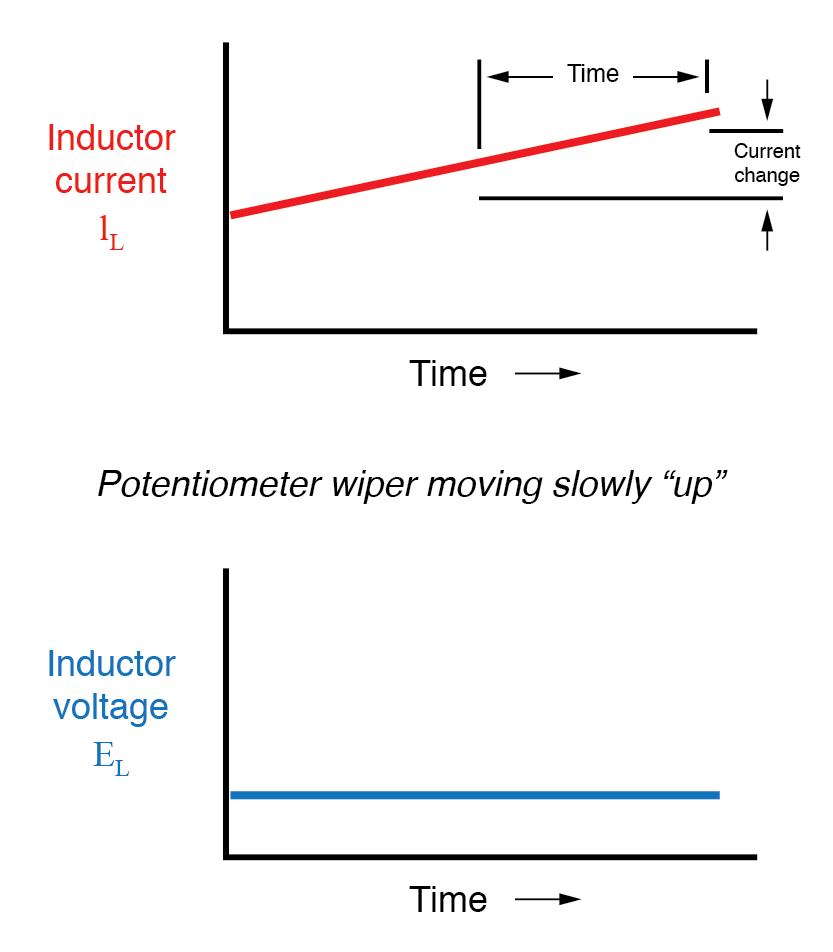Changing the rate of current increase through the inductor by moving the potentiometer wiper “up” at different speeds results in different amounts of voltage being dropped across the inductor, all with the same polarity (opposing the increase in current):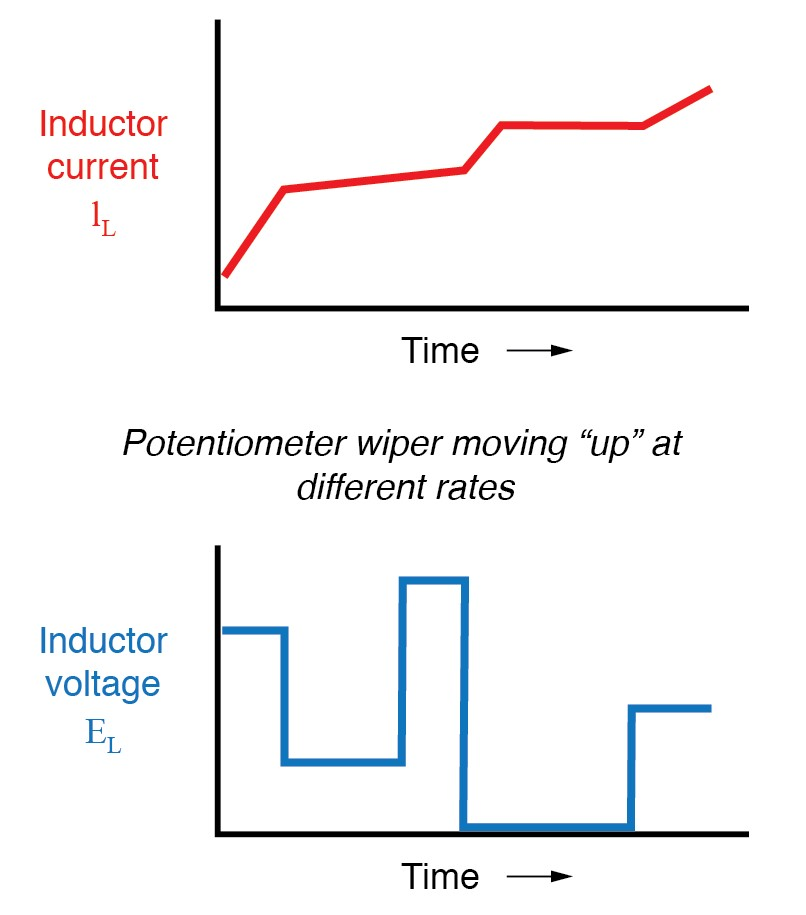Here again we see the derivative function of calculus exhibited in the behavior of an inductor. In calculus terms, we would say that the induced voltage across the inductor is the derivative of the current through the inductor: that is, proportional to the current’s rate-of-change with respect to time.

Reversing the direction of wiper motion on the potentiometer (going “down” rather than “up”) will result in its end-to-end resistance increasing. This will result in circuit current decreasing (a negative figure for di/dt). The inductor, always opposing any change in current, will produce a voltage drop opposed to the direction of change: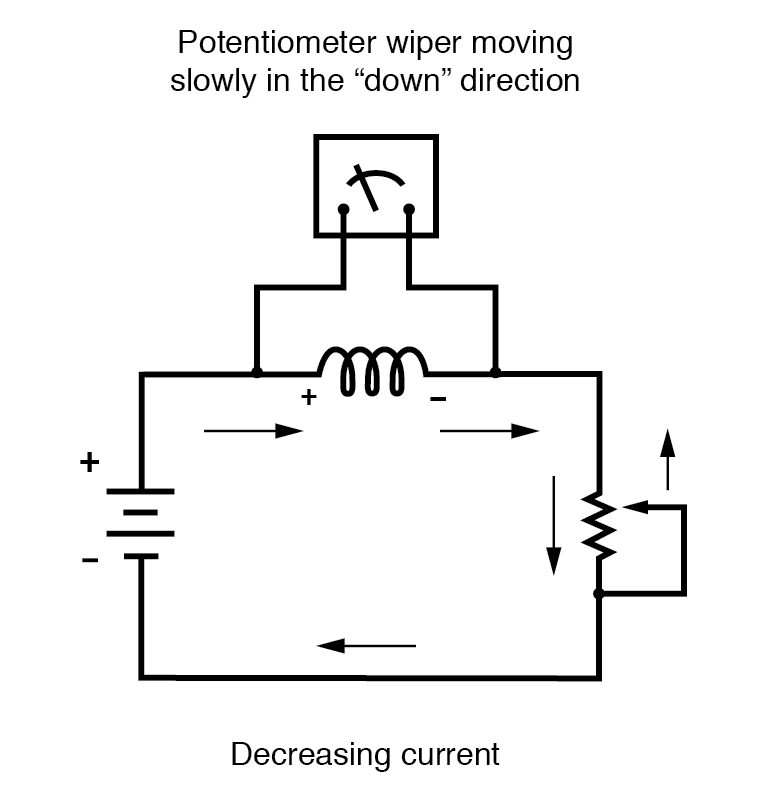How much voltage the inductor will produce depends, of course, on how rapidly the current through it is decreased. As described by Lenz’s Law, the induced voltage will be opposed to the change in current. With a decreasing current, the voltage polarity will be oriented so as to try to keep the current at its former magnitude. In this scenario, the inductor will be acting as a source, with the negative side of the induced voltage on the end where electrons are exiting, and the positive side of the induced voltage on the end where electrons are entering. The more rapidly current is decreased, the more voltage will be produced by the inductor, in its release of stored energy to try to keep current constant.

Again, the amount of voltage across a perfect inductor is directly proportional to the rate of current change through it. The only difference between the effects of a decreasing current and an increasing current is the polarity of the induced voltage. For the same rate of current change over time, either increasing or decreasing, the voltage magnitude (volts) will be the same. For example, a di/dt of -2 amps per second will produce the same amount of induced voltage drop across an inductor as a di/dt of +2 amps per second, just in the opposite polarity.

If current through an inductor is forced to change very rapidly, very high voltages will be produced. Consider the following circuit: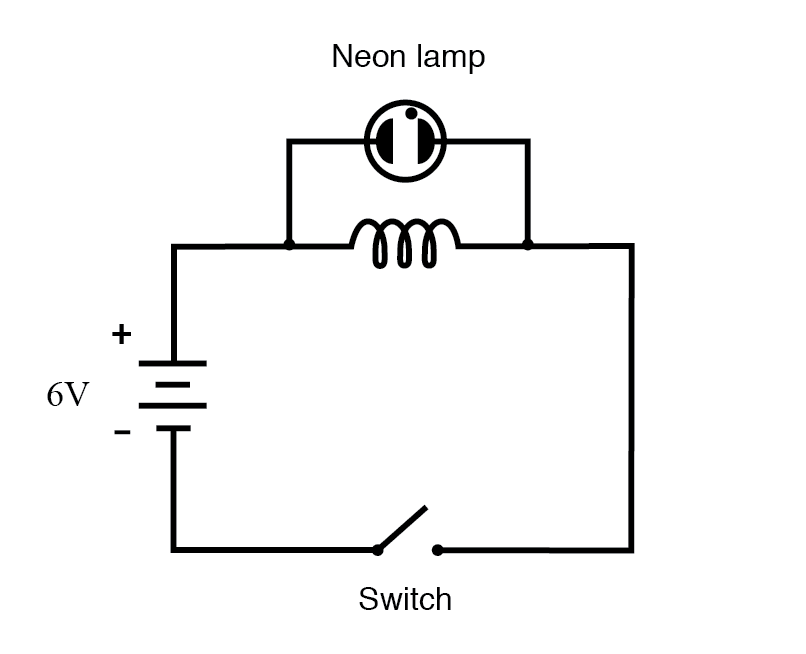In this circuit, a lamp is connected across the terminals of an inductor. A switch is used to control current in the circuit, and power is supplied by a 6 volt battery. When the switch is closed, the inductor will briefly oppose the change in current from zero to some magnitude, but will drop only a small amount of voltage. It takes about 70 volts to ionize the neon gas inside a neon bulb like this, so the bulb cannot be lit on the 6 volts produced by the battery, or the low voltage momentarily dropped by the inductor when the switch is closed: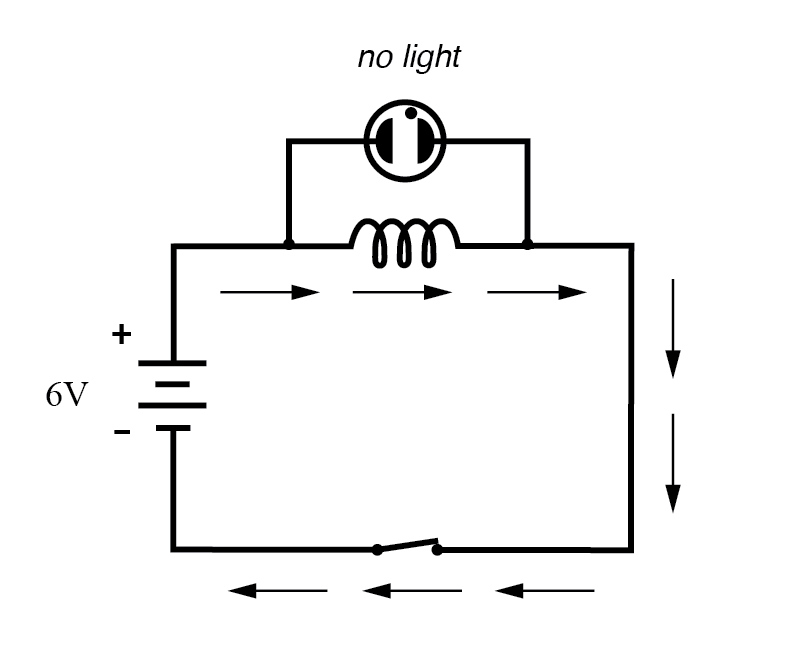When the switch is opened, however, it suddenly introduces an extremely high resistance into the circuit (the resistance of the air gap between the contacts). This sudden introduction of high resistance into the circuit causes the circuit current to decrease almost instantly. Mathematically, the di/dt term will be a very large negative number. Such a rapid change of current (from some magnitude to zero in very little time) will induce a very high voltage across the inductor, oriented with negative on the left and positive on the right, in an effort to oppose this decrease in current. The voltage produced is usually more than enough to light the neon lamp, if only for a brief moment until the current decays to zero: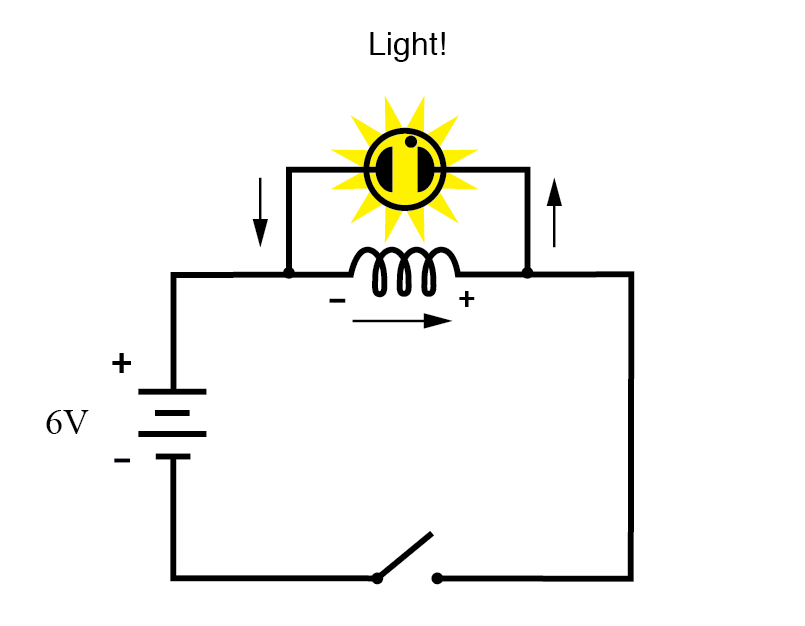For maximum effect, the inductor should be sized as large as possible (at least 1 Henry of inductance).

RELATED WORKSHEETS:

• Share
Published under the terms and conditions of the Design Science License
1 Comment• S
soncu August 02, 2020

Picture that is written under: Potantiometer wiper moving “up” at different rates, Vertical line says “Inductor Voltage (1L)” should have been : “Inductor current (1L)”

Like.